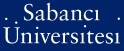# Transfinite diameter, Chebyshev constants, and capacities in C-n

Zakharyuta, Vyacheslav (2012) Transfinite diameter, Chebyshev constants, and capacities in C-n. Annales Polonici Mathematici, 106 . pp. 293-313. ISSN 0066-2216 (Print) 1730-6272 (Online)

Full text not available from this repository.

Official URL: http://dx.doi.org/10.4064/ap106-0-23

## Abstract

The famous result of geometric complex analysis, due to Fekete and Szego, states that the transfinite diameter d(K), characterizing the asymptotic size of K, the Chebyshev constant tau(K), characterizing the minimal uniform deviation of a monic polynomial on K and the capacity c(K), describing the asymptotic behavior of the Green function g(K)(z) at infinity, coincide. In this paper we give a survey of results on multidimensional notions of transfinite diameter, Chebyshev constants and capacities, related to these classical results and initiated by Leja's definition of transfinite diameter of a compact set K subset of C-n and the author's paper [Mat. Sb. 25 (1975)], where a multidimensional analog of the Fekete equality d(K) = tau(K) was first considered for any compact set in C-n. Using some general approach, we introduce an alternative definition of transfinite diameter and show its coincidence with Fekete Leja's transfinite diameter. In conclusion we discuss an application of the results of the author's paper mentioned above to the asymptotics of the leading coefficients of orthogonal polynomial bases in Hilbert spaces related to a given pluriregular polynomially convex compact set in C-n.

Item Type: Article transfinite diameter; Chebyshev constants; pluripotential Green functions; capacities; Vandermondian; Wronskian Q Science > QA Mathematics 21653 Vyacheslav Zakharyuta 02 Jul 2013 11:01 01 Aug 2019 10:35

Repository Staff Only: item control page